Note: The other languages of the website are Google-translated. Back to English

## How to multiply a range of cells by same number in Excel?

Supposing you have a range of numbers, and now you want to multiply them by a number 8.7, do you have any effective and quick methods to deal with this task?

#### Multiply a range cells by the same number with Paste Special function

Excel provides a quick way to apply a mathematical operation on a range of cells. You can use the Paste Special function to multiply a range of cells by a number as follows:

1. Input the number 8.7 into a blank cell and copy it.

2. Select the range that you want to multiply a value, and click Home > Paste > Paste Special. See screenshot below: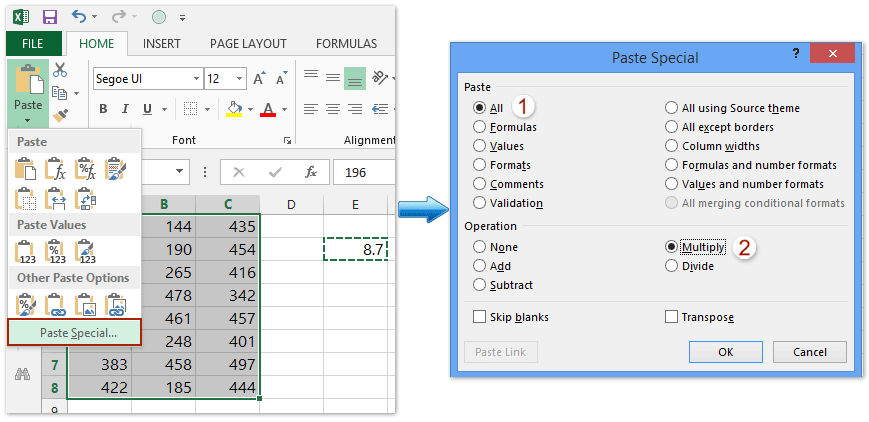3. In the Paste Special dialog box, click All option in the Paste section, and click Multiply option in the Operation section, and click the OK button. See screenshot above:

And the selected range will be multiplied by the number 8.7. See screenshot below: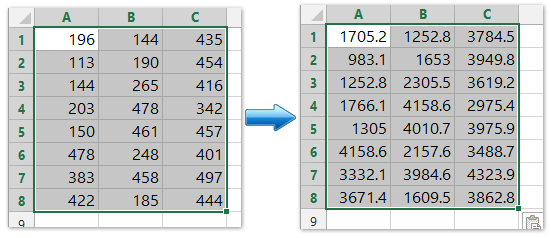#### Multiply a range cells by the same number with formula in Excel

Apart from the Paste Special feature, we can also apply a formula to Multiply a range of cells with the same number in Excel. Please do as follows:

1. Select a blank cell, says Cell E1, and type the formula =A1*\$D\$1 (A1 is the first cell of the range you will multiply with the same number, D1 is the cell with the specified number you will multiply with) into it, and press the Enter key.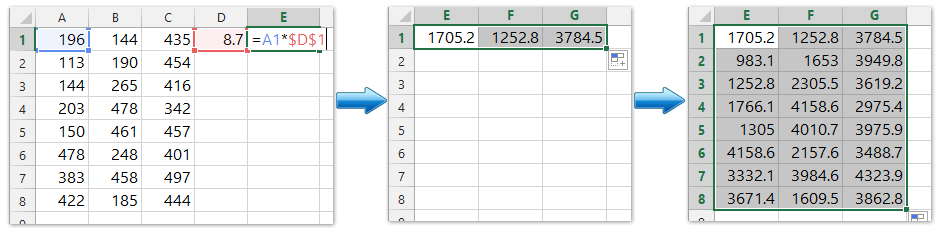2. Drag Cell E1's AutoFill handle right to Cell G1, and then drag down to the Cell G8. See screenshot above.

And now each cell in the specified range (A1:C8 in our case) are multiplied by 8.7 at once.

#### Multiply a range cells by the same number with/without formula

The Operations feature of Kutools for Excel can quickly apply various kinds of mathematical operations to a range of cells without using a formula. You can use it to multiply a range of cells by a number as follows.

Kutools for Excel - Includes more than 300 handy tools for Excel. Full feature free trial 30-day, no credit card required! Free Trial Now!

1. Select the range that you want to multiply a value, and click Kutools > More > Operation, see screenshot: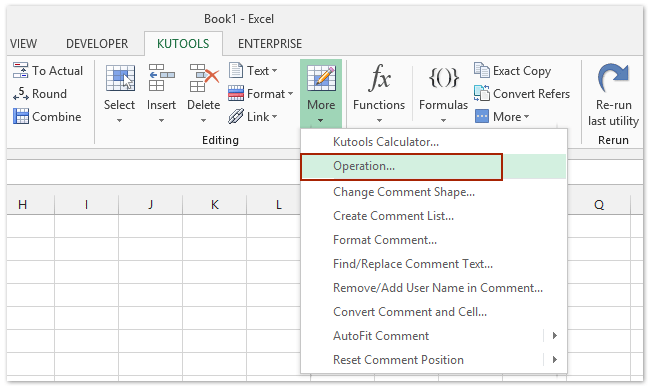2. In the Operation Tools dialog box, choose Multiplication from Operation box, and enter the number 8.7 into the Operand box, and click the Ok button. See screenshot: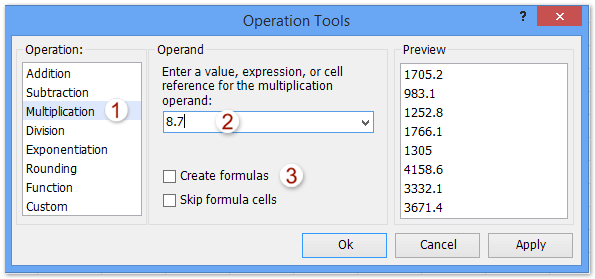Note: If you want to create formulas as well, you can check Create formulas option. If the selected cells include formulas, and you don’t want to multiply the calculated results of formulas, please check Skip formula cells option. See screenshot: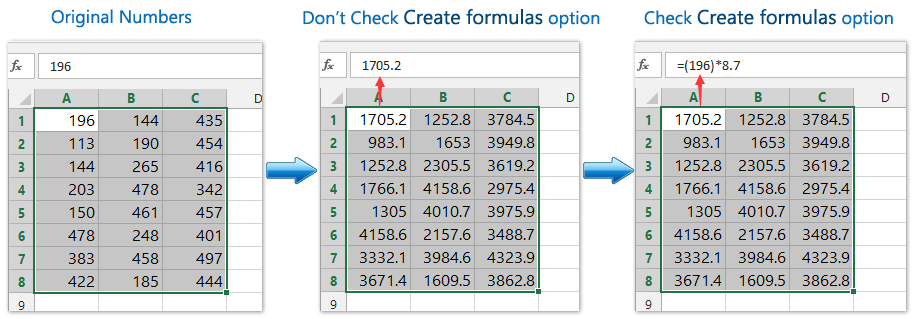#### Demo: Multiply a range cells by the same number with/without formula

Kutools for Excel includes more than 300 handy tools for Excel, free to try without limitation in 30 days. Download and Free Trial Now!

#### One click to convert multiple time to hours/minutes/seconds with automatically multiplying 24/1440/86400

For example you have a time of 2:23:42, you can convert this time to hours by multiplying 24 (or multiplying 1440 to minutes, multiplying 86400 to seconds; in other words, we can apply the formula =F2*24 for converting the time to hours, =F2*1440 to minutes, =F2*86400 to seconds), and then change the formula cell to Number format. But, with Kutools for Excel’s Convert Time utility, you can one click to batch convert multiple time data to hours/minutes/seconds only in Excel. Full Feature Free Trial 30-day!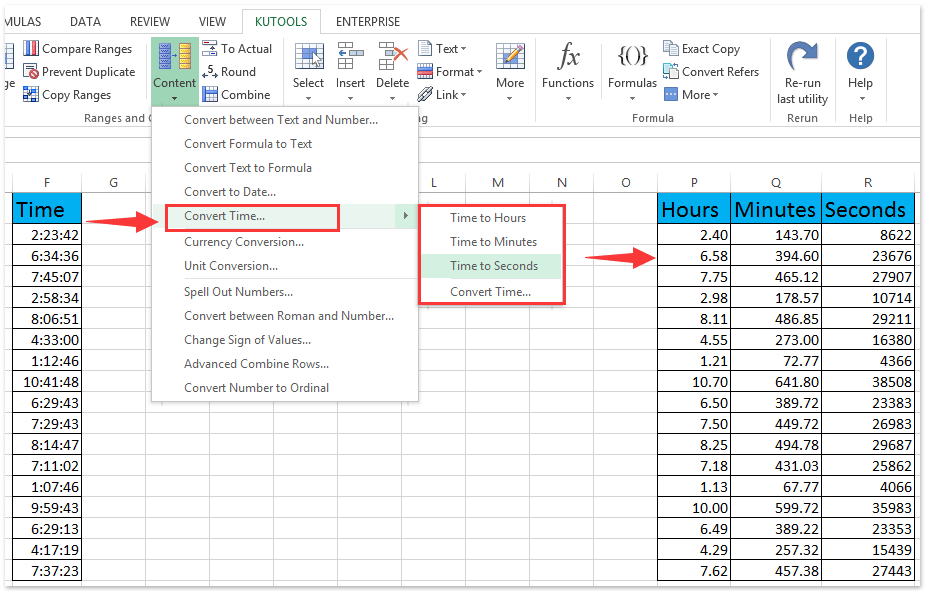#### Easily convert currencies by automatically multiplying the latest exchange rate in Excel

In general, we need to get the latest exchange rate between the two specified currencies, and then multiply the latest exchange rate to convert the original currency to the given currency. But, with Kutools for Excel's Currencies Conversion utility, it can automatically multiplying the latest exchange rate for easily batch converting currencies in Excel. Full Feature Free Trial 30-day!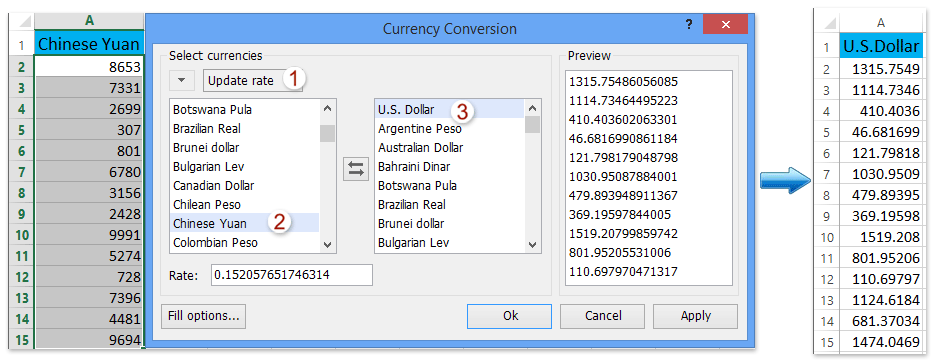### The Best Office Productivity Tools

#### Kutools for Excel Solves Most of Your Problems, and Increases Your Productivity by 80%

• Reuse: Quickly insert complex formulas, charts and anything that you have used before; Encrypt Cells with password; Create Mailing List and send emails...
• Super Formula Bar (easily edit multiple lines of text and formula); Reading Layout (easily read and edit large numbers of cells); Paste to Filtered Range...
• Merge Cells/Rows/Columns without losing Data; Split Cells Content; Combine Duplicate Rows/Columns... Prevent Duplicate Cells; Compare Ranges...
• Select Duplicate or Unique Rows; Select Blank Rows (all cells are empty); Super Find and Fuzzy Find in Many Workbooks; Random Select...
• Exact Copy Multiple Cells without changing formula reference; Auto Create References to Multiple Sheets; Insert Bullets, Check Boxes and more...
• Extract Text, Add Text, Remove by Position, Remove Space; Create and Print Paging Subtotals; Convert Between Cells Content and Comments...
• Super Filter (save and apply filter schemes to other sheets); Advanced Sort by month/week/day, frequency and more; Special Filter by bold, italic...
• Combine Workbooks and WorkSheets; Merge Tables based on key columns; Split Data into Multiple Sheets; Batch Convert xls, xlsx and PDF...
• More than 300 powerful features. Supports Office/Excel 2007-2019 and 365. Supports all languages. Easy deploying in your enterprise or organization. Full features 30-day free trial. 60-day money back guarantee.#### Office Tab Brings Tabbed interface to Office, and Make Your Work Much Easier

• Enable tabbed editing and reading in Word, Excel, PowerPoint, Publisher, Access, Visio and Project.
• Open and create multiple documents in new tabs of the same window, rather than in new windows.
• Increases your productivity by 50%, and reduces hundreds of mouse clicks for you every day!No ratings yet. Be the first to rate!
This comment was minimized by the moderator on the site
I am trying to create a formula for a cell and keep getting an error. I want to multiply a cell but i want it to figure different amounts. example: cell A19 i want it to figure cell A17 total if less than 500 multiply cell A18*5, then If cell A17 total is less than 1000 multiply A18*10, then if A17 is less than 1500 multiply A18*15, then if A17 is greater than 1500 multiply A18*20.what i have tried is: IF=(a17
This comment was minimized by the moderator on the site
I am trying to create a formula for a cell and keep getting an error. I want to multiply a cell but i want it to figure different amounts. example: cell A19 i want it to figure cell A17 total if less than 500 multiply cell A18*5, then If cell A17 total is less than 1000 multiply A18*10, then if A17 is less than 1500 multiply A18*15, then if A17 is greater than 1500 multiply A18*20.what i have tried is: IF=(a17[/quote]
This comment was minimized by the moderator on the site
:D Greate help, Thanks.
This comment was minimized by the moderator on the site
Thank you so much. This saved me a ton of time. :-)
This comment was minimized by the moderator on the site
Thank you so much! it was really helpful :)
This comment was minimized by the moderator on the site
hi hellow please can you help ya excel multiply formula could help with me please.
This comment was minimized by the moderator on the site
In Excel 2010 In 1. Cell Value is 12*4 In 2. Cell Value is 60 We want multiply 1*2 Cell each other and answer is 2880, how it is possible, please help.
This comment was minimized by the moderator on the site
after entering values in cell 1 (12/4) u have to copy and paste special that value in another cell b1

later u can multiply a2 with b1 and get ur result.
This comment was minimized by the moderator on the site
Let there are eight nos , 234 269 311 634 711 748 987 1001 Now I want to multiply the maximum of close numbers( the nos which are very close to each other) by a no. for example: 311 x A1, 748 x A2, & 1001 x A3 how should I proceed ?
This comment was minimized by the moderator on the site
in excel 2007
cell A1 value is 4
cell A2 value is 5
cell A3 value is 4
cell A4 value is 3

cell B5 value is 50

i want to multiply each cell multiply by B5.

Answer will only in C5 cell, even i change each value of cell A1 to A4.

for example if i change A3 value 4 to 6, answer will 50*6=300 etc.
This comment was minimized by the moderator on the site
may I know the answer for this? thank you
This comment was minimized by the moderator on the site
Hi Farhan,
1. Select Cell B5, press Ctrl + C to copy it。
2. Select Range A1:A4, right click, and click Paste Special > Paste Special in the context menu.
3. In the Paste Special dialog, check Multiply option, and click OK.
This comment was minimized by the moderator on the site
Great, thank you very much
There are no comments posted here yet